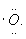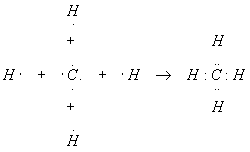Elementary Chemical Reactions

Here we cover some elementary concepts and study a few common chemical reactions. We are mostly interested in learning a simple explanation why the reactions happen at all, and how they happen when they do.

Atoms are stable, ordered collections of more fundamental particles (see the article on Quantum Mechanics for details): neutrons (0 charge, mass = 1 atomic mass unit = 1 AMU), electrons (-1 charge, mass = 1/1836 AMU) and protons (+1 charge, mass = 1 AMU). In an atom, the protons and neutrons are densely packed in the very small center of the atom, called the nucleus, while the electrons fly rapidly around the nucleus in the electronic orbitals or clouds. The electron cloud takes up most of the volume of an atom. Most of the mass of the electron is contained in the nucleus.

An atom is the smallest possible unit of a chemical element, a fundamental, individual substance. All stable matter in the universe consists of chemical elements and combinations of elements called chemical compounds.

The number of protons (in the nucleus) distinguishes atoms of the various chemical elements from each other. In fact, every element has an atomic number that is exactly the number of protons in one atom of that element. The atomic number is the single most important property of a chemical element, since the atomic number gives the identity of the element.

The second most important property of an element is its atomic mass. Naively, adding the number of protons, neutrons and electrons each times the mass of the particle (given above) computes this number. In fact, there is a slight discrepancy – the real atomic mass as measured by very precise experiments is always slightly lower than the sum of its parts. This mass deficit is caused by conversion of some of the mass of the atom to binding energy. The safest way to get the atomic mass for an element is read it off a periodic table of the elements. This is the familiar table found on the wall of almost every chemistry classroom in the world. Very nice copies (8.5 x 11 inches – notebook paper size) are readily available for a low price.

Most chemistry textbooks feature a periodic table printed on the inside front cover of the book.

Atoms must be electronically neutral in their pure state. Since like charges repulse each other with great force, and any charge on an atom would be repeated on every atom, a pure sample of an element with any charge at all would explode violently. In order for an atom to be neutral (zero charge), every proton (+1) must be matched with an electron (-1). Thus, there is an atom with 1 electron (and one proton), hydrogen (H), one with two, helium (He), three, lithium (Li), four, beryllium (Be), and so on up to about element 92, uranium (U). (Beyond element 92, the nuclei of the heavy elements are unstable, and so these heavy elements are not found naturally.)

Elements with certain "magic" numbers of electrons (2, 10, 18, 36, 54 and 86) are so stable, the elements do not react chemically. These elements are called the noble (or inert) gases. All other elements react with other elements to form compounds. In the special case where the reaction produces fixed numbers of the same types of atoms in exactly the same configuration, we say the elements have formed a molecule. Molecules are the simplest possible samples of new substances called molecular compounds. Usually, molecules form so that the atoms within them share electrons and thereby attain a magic number of electrons, with enhanced stability similar to the noble gases.

Although the orbitals that are occupied by the electrons in an atom are quite complicated to describe exactly, we can think of them as concentric shells around the nucleus. Each successive shell starting from the nucleus and working outward can contain only so many electrons. Once a shell is filled, additional electrons must occupy shells further out from the nucleus. The first shell can take only two electrons, the second 8, the third 8, the fourth 18, the fifth 18, and the sixth 32. Shells seven and above contain elements which are unstable. We have a simple rule for the reactions we study: apart from hydrogen, which is trying to get two electrons and so look like helium, all elements are trying to achieve eight electrons in their outer shell. Needless to say, this rule has many exceptions, but so many reactions obey it that we will consider it our cardinal rule for reactions.

Example

Atomic hydrogen is rarely found, but molecular hydrogen is common. Explain why.

Solution

Hydrogen is the first element, has one proton in the nucleus and one electron flying around. The first stable electron configuration is two electrons (looks like the first noble gas, helium). This, if two hydrogen atoms react:the result is that each hydrogen atom shares its electron with the other, and so both now have two electrons.  This molecule is much more stable (less reactive) than the two monatomic hydrogen atoms, so the reaction is strongly favored should two hydrogen atoms collide.

Example

The most abundant molecular substance on the surface of the earth is water, H2O. Explain why.

Solution

There is certainly a lot of oxygen near the surface of the earth, since the atmosphere is 21% oxygen. However, there is little free hydrogen. The atmosphere contains some, but not nearly as much as it does oxygen. On the other hand, interstellar space contains mostly free hydrogen, albeit very rarified. Where did all of earth’s hydrogen go? The simple answer is that most of it reacted with oxygen to form water.

Before we go into the reaction, we should take a look at oxygen. The atomic number of oxygen is 8, so oxygen has 8 electrons. The first two electrons complete the inner shell, so we are interested in the last six electrons in the outer shell. Just considering these, oxygen looks like this:Note that we have two pairs of electrons and two unpaired electrons. We can complete the set of eight electrons for oxygen by reacting it with two hydrogen atoms:Now let’s look at what we have. Each hydrogen atom has two (shared) electrons, so the first shell of electrons is filled for each H atom. These atoms now look like helium, and so they are "happy". The oxygen atom has eight electrons (in its second shell – we have ignored the two electrons in its inner shell since this shell is complete) due in part to sharing, so the second shell is filled. Oxygen now looks like neon, the next noble gas. All the atoms now have noble gas configuration, so this is a stable molecule.

Let’s review our fundamental rule for reactions. All elements, apart from the noble gases, are chemically reactive, because they are more stable electronically when they share electrons with other atoms. When this happens, the outer shell of electrons in each atom forms a magic number. Another example will be helpful here.

Example

One atom of carbon reacts with four hydrogen atoms to form methane, CH4. Show how this reaction works.

Solution

We already know what atomic hydrogen looks like, so let’s take a closer look at the carbon atom. The atomic number of carbon is 6, so carbon has six electrons. As was the case with oxygen above, the first two electrons complete the innermost shell, so we will ignore them and concentrate on the outer four electrons. The outer shell of carbon looks like this:To complete this shell, we need four more electrons, which is why carbon reacts with four hydrogen atoms. The hydrogen atoms contribute one electron each to the shared group, giving the carbon eight total electrons and a noble gas configuration. In the process, each hydrogen atom also shares one of the carbon four electrons, and so has two, completing its inner shell.The astute reader should have noticed that there seems to be something important about pairs of electrons. In the above examples, there was a pair of electrons between two different atoms. This shared pair of electrons is called a chemical bond. We will spend considerable space studying chemical bonds, since understanding bonding is key to understanding chemistry. In addition to bonds, we will also be interested in pairs of electrons not involved in bonding (the oxygen in water above has two of these), called lone pairs.

Example

A nitrogen atom reacts with three hydrogen atoms to form ammonia, NH3. Show how this reaction happens.

Solution

Nitrogen has atomic number 7 and so has seven electrons. The first two electrons fill the inner shell, leaving five for the outer shell. Thus, the outer shell of nitrogen looks like this:Completing the outer shell of electrons will evidently take three electrons, to be supplied by the three hydrogens. The reaction looks like this:This reaction produced three chemical bonds and one lone pair.

In short, describing chemical reactions as we have done reduces to a game: complete the shell. We will do additional examples in the next article to really nail this concept down. After the game of complete the shell has been mastered, we will start considering some of the many exceptions to this game and explore some of the consequences of violating our fundamental rule.